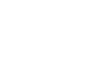What is gravitational force? - letsdiskussVanisha Anand

Student | Posted | Science-Technology

# What is gravitational force?

0
0

student | Posted

Gravity which is also known as gravitation is the universal force which acts as an attraction between all matters. This force is the weakest force known by now. So, it thus does not play any role which determines any internal properties of everyday working. If the objects are smaller than their gravitational force is stronger and vice versa. The term gravity was given by Isaac Newton. This discovery gave science a new and better understanding of planetary motion.0
0